# PSAT Math : How to find the area of a circle

## Example Questions

### Example Question #28 : Radius

If the radius of Circle A is three times the radius of Circle B, what is the ratio of the area of Circle A to the area of Circle B?

12

6

3

9

15

9

Explanation:

We know that the equation for the area of a circle is π r2. To solve this problem, we pick radii for Circles A and B, making sure that Circle A’s radius is three times Circle B’s radius, as the problem specifies. Then we will divide the resulting areas of the two circles. For example, if we say that Circle A has radius 6 and Circle B has radius 2, then the ratio of the area of Circle A to B is: (π 62)/(π 22) = 36π/4π. From here, the π's cancel out, leaving 36/4 = 9.

### Example Question #541 : Act Math

1. A circle is inscribed inside a 10 by 10 square. What is the area of the circle?

10π

100π

40π

25π

50π

25π

Explanation:

Area of a circle = A = πr2

R = 1/2d = ½(10) = 5

A = 52π = 25π

### Example Question #11 : Plane Geometry

A square has an area of 1089 in2. If a circle is inscribed within the square, what is its area?

33π in2

272.25π in2

1089π in2

16.5 in2

33 in2

272.25π in2

Explanation:

The diameter of the circle is the length of a side of the square. Therefore, first solve for the length of the square's sides. The area of the square is:

A = s2 or 1089 = s2. Taking the square root of both sides, we get: s = 33.

Now, based on this, we know that 2r = 33 or r = 16.5. The area of the circle is πr2 or π16.52 = 272.25π.

### Example Question #311 : Sat Mathematics

A square has an area of 32 in2. If a circle is inscribed within the square, what is its area?

16π in2

8π in2

4√2 in2

2√2 in2

32π in2

8π in2

Explanation:

The diameter of the circle is the length of a side of the square. Therefore, first solve for the length of the square's sides. The area of the square is:

A = s2 or 32 = s2. Taking the square root of both sides, we get: s = √32 = √(25) = 4√2.

Now, based on this, we know that 2r = 4√2 or r = 2√2. The area of the circle is πr2 or π(2√2)2 = 4 * 2π = 8π.

A manufacturer makes wooden circles out of square blocks of wood. If the wood costs $0.25 per square inch, what is the minimum waste cost possible for cutting a circle with a radius of 44 in.? Possible Answers: 1936 – 484π dollars 5808 dollars 1936π dollars 7744 – 1936π dollars 1936 dollars Correct answer: 1936 – 484π dollars Explanation: The smallest block from which a circle could be made would be a square that perfectly matches the diameter of the given circle. (This is presuming we have perfectly calibrated equipment.) Such a square would have dimensions equal to the diameter of the circle, meaning it would have sides of 88 inches for our problem. Its total area would be 88 * 88 or 7744 in2. Now, the waste amount would be the "corners" remaining after the circle was cut. The area of the circle is πr2 or π * 442 = 1936π in2. Therefore, the area remaining would be 7744 – 1936π. The cost of the waste would be 0.25 * (7744 – 1936π). This is not an option for our answers, so let us simplify a bit. We can factor out a common 4 from our subtraction. This would give us: 0.25 * 4 * (1936 – 484π). Since 0.25 is equal to 1/4, 0.25 * 4 = 1. Therefore, our final answer is: 1936 – 484π dollars. ### Example Question #84 : Circles A manufacturer makes wooden circles out of square blocks of wood. If the wood costs$0.20 per square inch, what is the minimum waste cost possible for cutting a circle with a radius of 25 in.?

500 dollars

2500 - 625π dollars

625 dollars

500 - 125π dollars

625 - 25π dollars

500 - 125π dollars

Explanation:

The smallest block from which a circle could be made would be a square that perfectly matches the diameter of the given circle. (This is presuming we have perfectly calibrated equipment.) Such a square would have dimensions equal to the diameter of the circle, meaning it would have sides of 50 inches for our problem. Its total area would be 50 * 50 or 2500 in2.

Now, the waste amount would be the "corners" remaining after the circle was cut. The area of the circle is πr2 or π * 252 = 625π in2. Therefore, the area remaining would be 2500 - 625π. The cost of the waste would be 0.2 * (2500 – 625π). This is not an option for our answers, so let us simplify a bit. We can factor out a common 5 from our subtraction. This would give us: 0.2 * 5 * (500 – 125π). Since 0.2 is equal to 1/5, 0.2 * 625 = 125. Therefore, our final answer is: 500 – 125π dollars.

### Example Question #11 : How To Find The Area Of A Circle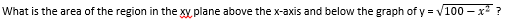25π

50π

10π

20π

100π

50π

Explanation: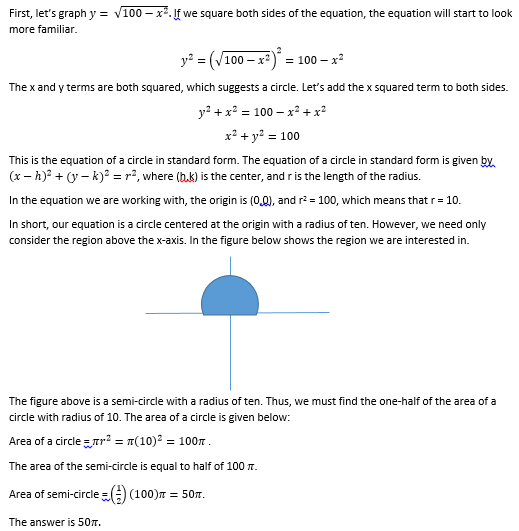### Example Question #86 : Circles

A circle with diameter of length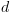is inscribed in a square. Which of the following is equivalent to the area inside of the square, but outside of the circle?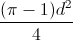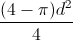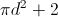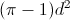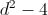Explanation:

In order to find the area that is inside the square but outside the circle, we will need to subtract the area of the circle from the area of the square. The area of a circle is equal to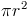. However, since we are given the length of the diameter, we will need to solve for the radius in terms of the diameter. Because the diameter of a circle is twice the length of its radius, we can write the following equation and solve for: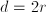Divide both sides by 2.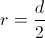We will now substitute this into the formula for the area of the circle.

area of circle =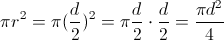We next will need to find the area of the square. Because the circle is inscribed in the square, the diameter of the circle is equal to the length of the circle's side. In other words, the square has side lengths equal to d. The area of any square is equal to the square of its side length. Therefore, the area of the square is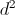.

area of square =Lastly, we will subtract the area of the circle from the area of the square.

difference in areas =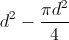We will rewriteso that its denominator is 4.

difference in areas =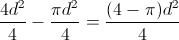The answer is.

### Example Question #87 : Circles

An original circle has an area of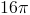. If the radius is increased by a factor of 3, what is the ratio of the new area to the old area?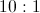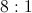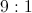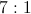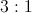Explanation:

The formula for the area of a circle is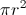. If we increase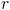by a factor of 3, we will increase the area by a factor of 9.

### Example Question #21 : How To Find The Area Of A Circle

A square has an area of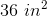.  If the side of the the square is the same as the diameter of a circle, what is the area of the circle?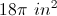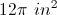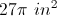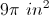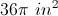The area of a square is given by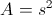so we know that the side of the square is 6 in.  If a circle has a diameter of 6 in, then the radius is 3 in.  So the area of the circle is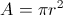or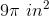.